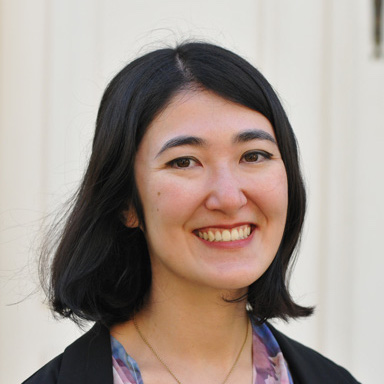# Global Optimality of Sparse Dictionary Learning with Applications to Subspace Clustering

### Abstract

Most signal processing applications require a good choice of representation of the data for numerous reasons. For instance, classical tasks such as compression, denoising or classification can be performed more easily when the data is represented in terms of certain bases that exploit special structure in high-dimensional data. One specific representation is obtained by decomposing the data in terms of a dictionary, whose elements are either predefined such as wavelets or learned directly from the data. When the representation is assumed to be sparse, i.e., when each data point is a linear combination of a few elements from the dictionary, the latter problem is called Sparse Dictionary Learning (SDL). This class of data modeling methods exploits certain structure in the data which can be formulated as: the data lies in a union of low-dimensional subspaces and therefore the dictionary elements, called atoms, can be seen as basis elements of these subspaces. Since the dictionary is typically overcomplete, the atoms are actually dependent and should be considered as frames for the subspaces that capture the main directions in terms of compact representations. While a variety of efficient algorithms that give approximate solutions for the SDL problem have been proposed, the theoretical analysis of this problem remains a key challenge due to its non-convexity. In this work we present a step towards a theoretical understanding of global optimality for the SDL problem. In particular, we show that by regularizing the SDL problem with a certain function that penalizes the number of dictionary atoms it is possible to derive conditions under which an optimal dictionary can be found. We also demonstrate this analysis with an application of SDL to the problem of Subspace Clustering.

Type
Date